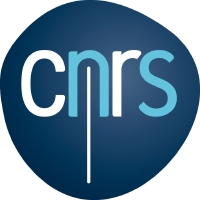## Michel Lassalle

Directeur de recherche émérite
Section de Mathématiques
Centre National de la Recherche Scientifique (CNRS)

Institut Gaspard Monge
Université de Marne-la-Vallée
F-77454 Marne-la-Vallée Cedex 2
France

List of publications

Some preprints

Tables for
characters of the
symmetric group

Symmetric functions
and
Jucys-Murphy elements

Tables for
Jack polynomials :

Jack polynomials
and alpha-contents

A conjecture for
Jack polynomials

Jack polynomials
and free cumulants
Research interests :

• Mathematical physics
Properties of n-point functions in general quantum field theory.
Exactly solvable quantum many-body systems (Calogero-Sutherland type).

• Groups and complex analysis
Harmonic analysis in complex homogeneous spaces :
Generalized Reinhardt domains, Hardy spaces, Cauchy and Poisson kernels.
Generalization of the tube and three-circle theorems.

• Hermitian symmetric spaces
Orbital structure, harmonic analysis, Hua equations, R-spaces.

• Jordan algebras
Formally real and complex Jordan algebras :
Inversible elements, Pierce decomposition.
Quasi-invariant measures on the cone of squares.

• Algebraic combinatorics
Symmetric functions, classical theory of partitions.
Jack polynomials, Macdonald polynomials.
Generalization of classical orthogonal polynomials (Hermite, Laguerre, Jacobi).
Generalized binomial coefficients.
Shifted Jack polynomials, shifted Macdonald polynomials.
Characters of the symmetric group.

• Free probability theory